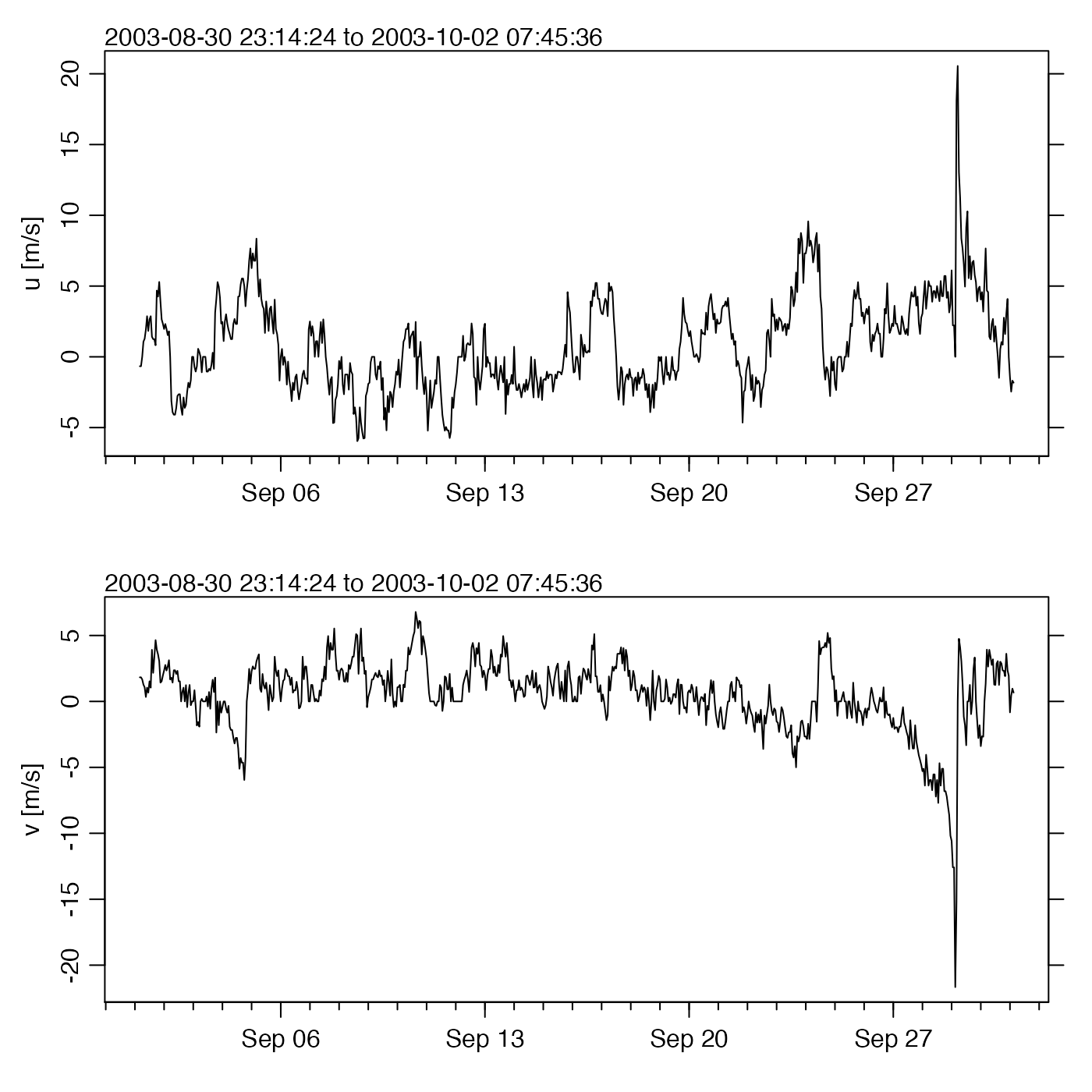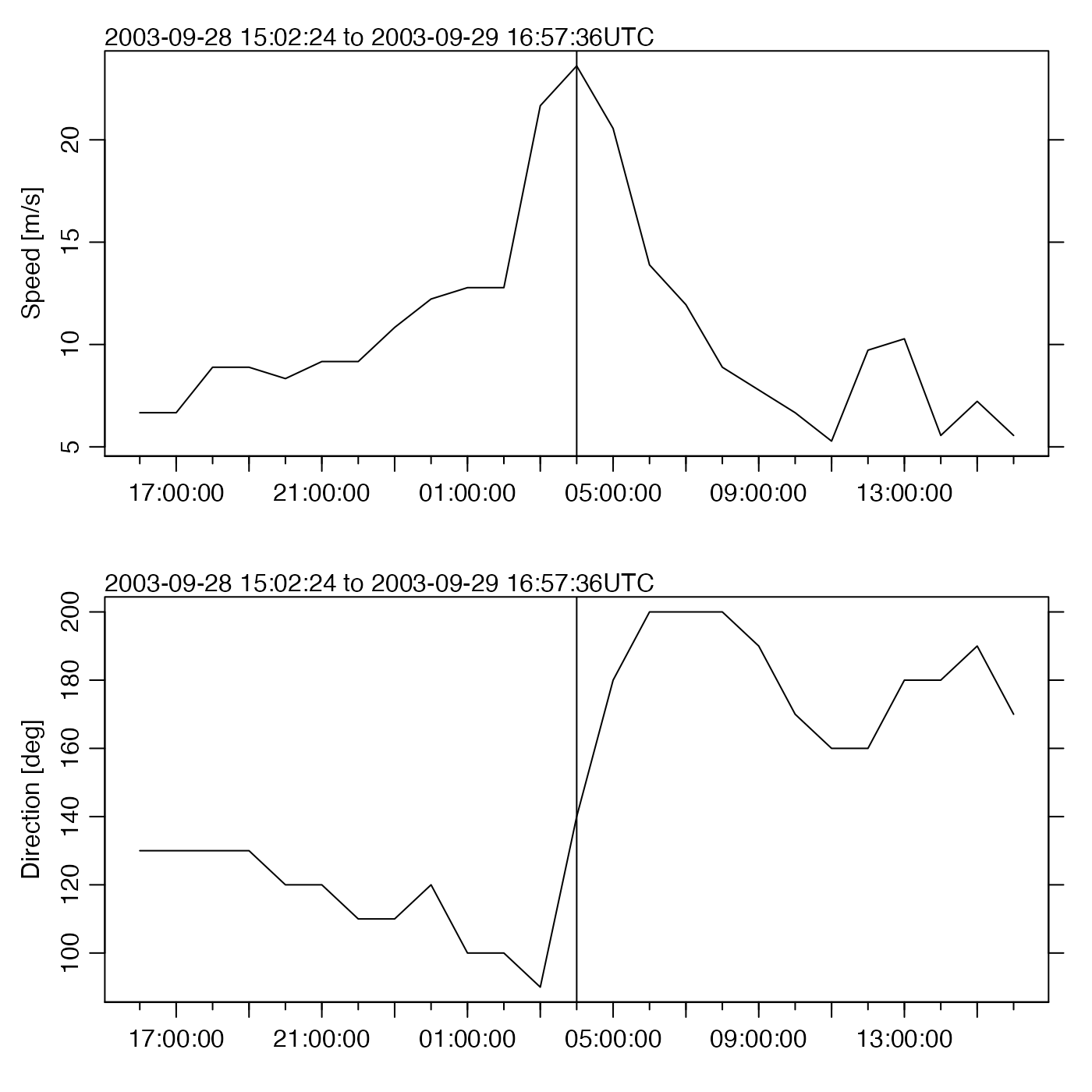Creates a multi-panel summary plot of data measured in a meteorological data set. cast. The panels are controlled by the which argument.

# S4 method for met
plot(x, which = 1:4, mgp, mar, tformat, debug = getOption("oceDebug"))

## Arguments

x

a met object.

which

list of desired plot types.

• which=1 gives a time-series plot of temperature

• which=2 gives a time-series plot of pressure

• which=3 gives a time-series plot of the x (eastward) component of velocity

• which=4 gives a time-series plot of the y (northward) component of velocity

• which=5 gives a time-series plot of speed

• which=6 gives a time-series plot of direction (degrees clockwise from north; note that the values returned by met[["direction"]] must be multiplied by 10 to get the direction plotted)

mgp

A 3-element numerical vector used with par("mgp") to control the spacing of axis elements. The default is tighter than the R default.

mar

A 4-element numerical vector used with par("mar") to control the plot margins. The default is tighter than the R default.

tformat

optional argument passed to oce.plot.ts(), for plot types that call that function. (See strptime() for the format used.)

debug

an integer specifying whether debugging information is to be printed during the processing. This is a general parameter that is used by many oce functions. Generally, setting debug=0 turns off the printing, while higher values suggest that more information be printed. If one function calls another, it usually reduces the value of debug first, so that a user can often obtain deeper debugging by specifying higher debug values.

## Details

If more than one panel is drawn, then on exit from plot.met, the value of par will be reset to the value it had before the function call. However, if only one panel is drawn, the adjustments to par made within plot.met are left in place, so that further additions may be made to the plot.

Other functions that plot oce data: download.amsr(), plot,adp-method, plot,adv-method, plot,amsr-method, plot,argo-method, plot,bremen-method, plot,cm-method, plot,coastline-method, plot,ctd-method, plot,gps-method, plot,ladp-method, plot,landsat-method, plot,lisst-method, plot,lobo-method, plot,odf-method, plot,rsk-method, plot,satellite-method, plot,sealevel-method, plot,section-method, plot,tidem-method, plot,topo-method, plot,windrose-method, plot,xbt-method, plotProfile(), plotScan(), plotTS(), tidem-class

Other things related to met data: [[,met-method, [[<-,met-method, as.met(), download.met(), met-class, met, read.met(), subset,met-method, summary,met-method

Dan Kelley

## Examples

library(oce)
data(met)
plot(met, which=3:4)# Wind speed and direction during Hurricane Juan
# Compare with the final figure in a white paper by Chris Fogarty
# (available at http://www.novaweather.net/Hurricane_Juan_files/McNabs_plot.pdf